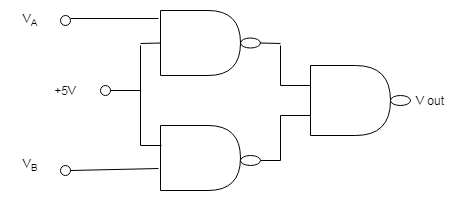Tribhuvan University

Institute of Science and Technology

2077

Bachelor Level / first-semester / Science

Computer Science and Information Technology( PHY113 )

Physics

Full Marks: 60 + 20 + 20

Pass Marks: 24 + 8 + 8

Time: 3 Hours

Candidates are required to give their answers in their own words as far as practicable.

The figures in the margin indicate full marks.

Group A

Attempt any two questions. (2 X 10 = 20)

1

Explain RTL and TTL  gates. How memory and clock circuits can be made by using these gates? Explain how they work?

2

Set up a differential equation for an oscillation of a spring using Hooke’s and Newton’s second law. Find the general solution of this equation and hence the expressions for the period, velocity, and acceleration of oscillation.

3

Describe the Frank-Hertz experiment. Discuss its result and outline limitations.

Group B

Attempt any Eight questions. (8 X 5 = 40)

4

Discuss magnetic dipole moment. What is its effect on atoms and on molecules? Explain.

5

Describe the following process of IC production: (a) Oxidation, (b) Pattern definition, and (c) Doping.

6

Explain the construction and working of bipolar junction transistor (BJT)

7

A roulette wheel with moment of inertia I = 0.5 kgm2 rotating initially at 2 rev/sec coasts to a stop from the constant friction torque of bearing. If the torque of the bearing. If the torque is 0.4 Nm, how long does it take to stop?

8

Two large parallel plates are separated by a distance of 5 cm. The plates have equal but opposite charges that create an electric field in the region between the plate. An α particle ( q = 3.2 × 10-27 kg) is released from the positively charged plate, and it strikes the negatively charged plate 2 × 10-6 second later. Assuming that the electric field between the plates is uniform and perpendicular to the plates. what is the strength of the electric field?

9

A neutron spectroscopy a beam of mono energetic neutrons is obtained by reflecting reactor neutrons from a beryllium crystal. If separation between the atomic planes of the beryllium crystal is 0.732 A°, what is the angle between the incident neutron beam and the atomic planes that will yield a monochromatic beam of neutrons of wavelength 0.1 A°.

10

What is the probability of finding a particle in a well of width ∝ at a position $$\frac{\alpha }{4}$$ from the wall if n = 1, if n = 2, if n = 3. Use the normalized wavefunction ψ(x,t) =$$\left ( \frac{2}{a} \right )^{\frac{1}{2}}$$ sin$$\left ( \frac{n\pi x}{a} \right )e^{-\frac{iEt}{h cut}}$$.

11

The energy gap in silicon is 1.1 eV, whereas in diamond it is 6eV. What conclusion can you draw about the transparency of the two materials to visible light(4000 A° to 7000 A°)?

12

Find the truth table for the circuit shown in the figure. What logic function will the circuit perform if the constant +5 V input to the first two gates is changed to ground potential?